Open in App
Not now

# Puzzle | Cut Blue Painted Cube

• Difficulty Level : Medium
• Last Updated : 18 Jan, 2023

A solid, n-inch cube of wood is coated with blue paint on all six sides.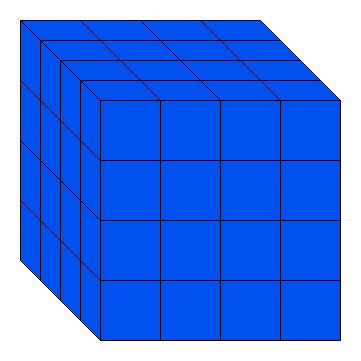Then the cube is cut into smaller one-inch cubes. These new one-inch cubes will have either Three blue sides, Two blue sides, One blue side, or No blue sides.

How many of each type 1 – inch cube will there be?

### Solution:

This puzzle can be solved logically or mathematically.

After cutting,
total no of cubes will be: n*n*n = n^3

Then,
Number of each type of cube can be found out by using the following formulas:

• 1 sided painted =6 * (n-2)^2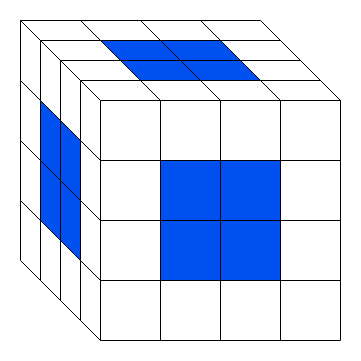• 2 sided painted = 12 *(n-2 )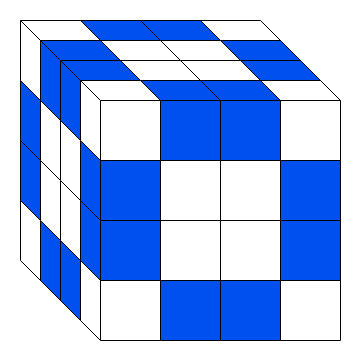• 3 sided painted = Always 8 (a cube has 8 corner)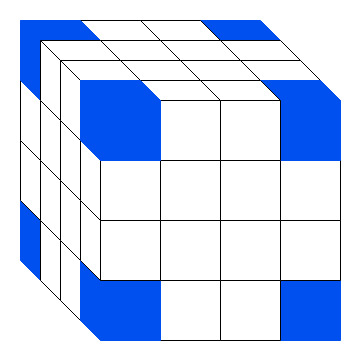• No sided painted =(n-2)^3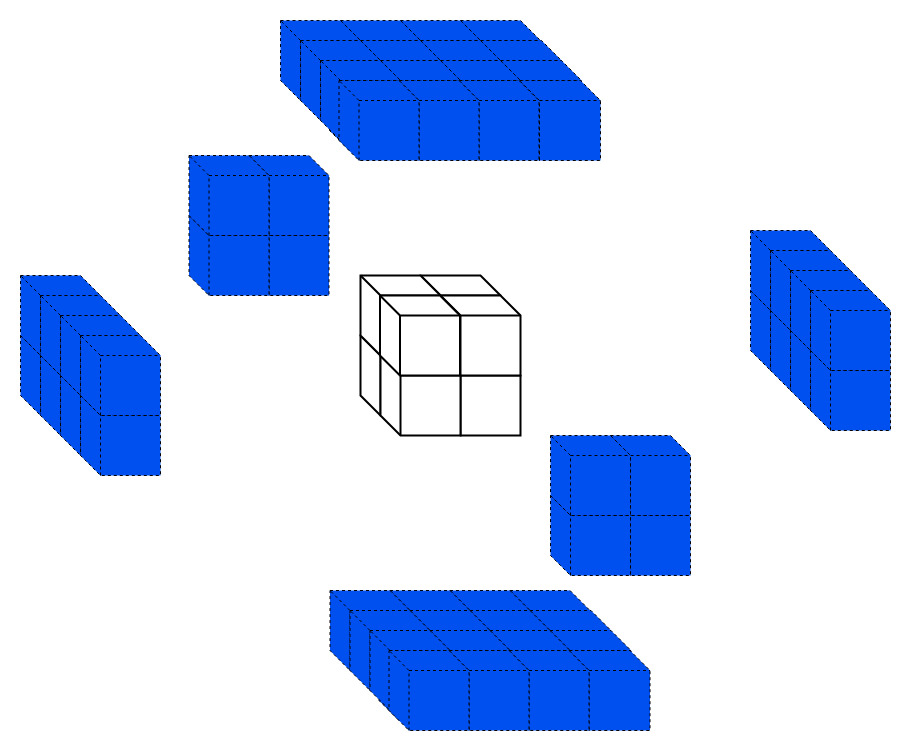### Examples:

Question 1:
A solid, 4-inch cube of wood is coated with blue paint on all six sides.Then the cube is cut into smaller one-inch cubes.These new one-inch cubes will have either Three blue sides, Two blue sides, One blue side, or No blue sides.then How many of each 1-inch cube will there be?

Solution:
given n=4 then
No sided painted =(4-2)^3 =>8
1 sided painted =6 * (n-2)^2 =>24
2 sided painted = 12 *(n-2 ) => 24
3 sided painted = Always 8 (a cube has 8 corner)

Question 2:
A solid, 8-inch cube of wood is coated with blue paint on all six sides.Then the cube is cut into smaller one-inch cubes.These new one-inch cubes will have either Three blue sides, Two blue sides, One blue side, or No blue sides.then How many of each 1-inch cube will there be?

Solution:
given n=8 then
No sided painted =(8-2)^3 =>216
1 sided painted =6 * (n-2)^2 =>216
2 sided painted = 12 *(n-2 ) => 72
3 sided painted = Always 8 (a cube has 8 corner)

Question 3:
when 64 inch cube cut into smaller 4 inch cube then n=given_size/cutting_size
for given question
n=64/4 =16
then
No sided painted =(16-2)^3 =>2744
1 sided painted =6 * (16-2)^2 =>1176
2 sided painted = 12 *(n-2 ) => 168
3 sided painted = Always 8 (a cube has 8 corner)

My Personal Notes arrow_drop_up
Related Articles